Courses

# Test: Data Handling - 3

## 20 Questions MCQ Test Mathematics (Maths) Class 7 | Test: Data Handling - 3

Description
This mock test of Test: Data Handling - 3 for Class 7 helps you for every Class 7 entrance exam. This contains 20 Multiple Choice Questions for Class 7 Test: Data Handling - 3 (mcq) to study with solutions a complete question bank. The solved questions answers in this Test: Data Handling - 3 quiz give you a good mix of easy questions and tough questions. Class 7 students definitely take this Test: Data Handling - 3 exercise for a better result in the exam. You can find other Test: Data Handling - 3 extra questions, long questions & short questions for Class 7 on EduRev as well by searching above.
QUESTION: 1

### What is the arithmetic mean of 1, 2,...., 9 and 10?

Solution:

By definition, A.M.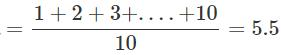By shortcut, A.M.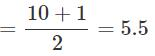QUESTION: 2

### In a data, 10 numbers are arranged in increasing order. If the 7th entry is increased by 4, by how much does the median increase?

Solution:

For 10 observations, the median would be the average of the 5th and 6th observations. Since they are unaffected by increase in 7th entry, the median will be unchanged.

QUESTION: 3

### What is the mean of x, x+3, x+6, x+9 and x+12?

Solution:

By definition, Average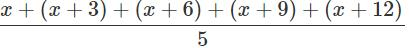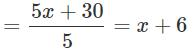QUESTION: 4

The daily sales of kerosene (in litres) in a ration shop for six days is given in the box.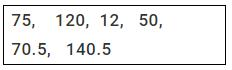What is the average daily sale?

Solution:

By definition of average, the average daily sale =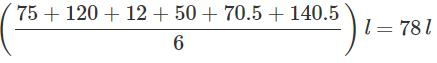However, without calculating we can say that the answer is D since the average lies between the maximum and the minimum.

QUESTION: 5

The mean of five numbers is 27. If one of the numbers is excluded, the mean gets reduced by 2. What is the excluded number?

Solution:

Let the sum of four numbers be y and the excluded number be x.
Then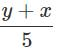=27  and , y/4 = 25 or x+y = 135 and y = 100 ⇒ x = 35

QUESTION: 6

What is the median of the data 46,64,87, 41,58,77,35,90,55,33,92?

Solution:

Arranging the given data in ascending order, we have, 33, 35, 41, 46, 55, 58, 64, 77, 87, 90 and 92. The sixth entry is 58.
∴ Median is 58.

QUESTION: 7

Which of the following is true about mean?

Solution:
QUESTION: 8

If each entry of a data is increased by 5, how does the mean change?

Solution:

Let new mean be M , no. of observations be n and old sum is x
So if every entry is increased by 5 sum of observations is increased by 5n
M=(s+5n)/n
=s/n + 5n/n
=s/n +5
So the new mean is increased by 5

QUESTION: 9

The arithmetic mean of five given numbers is 85. What is their sum?

Solution:

arithmetic mean = sum of observation / total no of observation

therefore;

sum of observation = arithmetic mean x total no of observation

sum of observation = 85 X 5=425

QUESTION: 10

The average weight of a sample of 10 apples is 52 g. Later it was found that the weighing machine had shown the weight of each apple 10 g less. What is the correct average weight of an apple?

Solution:

Since every apple has now 10g more, the average will rise by 10g, So accurate average= 52+10=62g

QUESTION: 11

The mean of 6, y, 7, x and 14 is 8.Which of the following is true?

Solution:

Mean=(6+y+7+x+14)/5
8=(27+y+x)/5
40=27+y+x
y+x=40-27=13

QUESTION: 12

Which of the following is correct about mode?

Solution:
QUESTION: 13

Rajani has a box with 6 marbles numbered from 1 to 6 on each of them. She picks a marble from it without seeing. What is the probability that the marble picked has the number 3 on it?

Solution:

Each of the 6 marbles has an equal chance of being picked. So, the probability that the marble picked is 3 will be 1 out of 6 i.e. 1/6.

QUESTION: 14

The heights of six mountains are 8200 m, 6000 m, 8600 m, 7500 m, 8800 m and 6500 m. Based on this information, answer the questions given.
Q. What is the approximate average height of the mountains?

Solution:

Average height of the mountains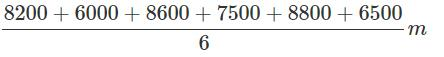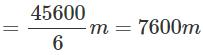QUESTION: 15

The heights of six mountains are 8200 m, 6000 m, 8600 m, 7500 m, 8800 m and 6500 m. Based on this information, answer the questions given. Find the median height of the mountains.

Solution:

Arranging the heights in ascending order, we have 6000, 6500, 7500, 8200, 8600, 8800.
∴  Median height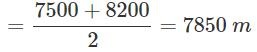QUESTION: 16

The heights of six mountains are 8200 m, 6000 m, 8600 m, 7500 m, 8800 m and 6500 m. Based on this information, answer the questions given.
Q. What is the mode of the heights?

Solution:
QUESTION: 17

The heights of six mountains are 8200 m, 6000 m, 8600 m, 7500 m, 8800 m and 6500 m. Based on this information, answer the questions given.
Q. Which of the following statements is true?

Solution:

Arranging in ascending order=6000,6500,7500,8200,8600,8800
Median=7500+8200/2=15700/2=7850
Mean=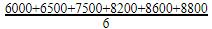= 7600
So, median height is greater than mean height.

QUESTION: 18

The heights of six mountains are 8200 m, 6000 m, 8600 m, 7500 m, 8800 m and 6500 m. Based on this information, answer the questions given. Rakesh and Sanjay planned to go trekking on any of these mountains. They wrote the heights on chits of paper, shuffled them and picked one.
Q. What is the probability that the height picked is the maximum?

Solution:
QUESTION: 19

The ages (in years) of some teachers of a school are given in the box. 26, 32,38, 41,26, 31, 35,33,26,37 Based on this information, answer the following questions.
Q. What is the range of the ages of the teachers?

Solution:
QUESTION: 20

The ages (in years) of some teachers of a school are given in the box. 26, 32,38, 41,26, 31, 35,33,26,37 Based on this information, answer the following questions.
Q. What is the mean age of the teachers?

Solution:

Mean =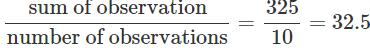years.